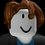# Repeating decimals.

We can use the fractions formula.

Like $\displaystyle 0.xxxxxxx\cdots = 0.\dot x = \frac x9$, but if $\displaystyle 0.99999999\cdots = 0.\dot 9 = \frac 99 = 1$.

And there are too many ways to prove that $\displaystyle 0.999999\cdots = 0.\dot 9 = 1$.

And it is not only for $0.xxxxxxx\cdots$.

It is almost for that $\displaystyle a.bbbbbbbbbbb\cdots = a.\dot b = \frac { ab - a } { 9 }$.Note by . .
4 months, 3 weeks ago

This discussion board is a place to discuss our Daily Challenges and the math and science related to those challenges. Explanations are more than just a solution — they should explain the steps and thinking strategies that you used to obtain the solution. Comments should further the discussion of math and science.

When posting on Brilliant:

• Use the emojis to react to an explanation, whether you're congratulating a job well done , or just really confused .
• Ask specific questions about the challenge or the steps in somebody's explanation. Well-posed questions can add a lot to the discussion, but posting "I don't understand!" doesn't help anyone.
• Try to contribute something new to the discussion, whether it is an extension, generalization or other idea related to the challenge.

MarkdownAppears as
*italics* or _italics_ italics
**bold** or __bold__ bold
- bulleted- list
• bulleted
• list
1. numbered2. list
1. numbered
2. list
Note: you must add a full line of space before and after lists for them to show up correctly
paragraph 1paragraph 2

paragraph 1

paragraph 2

[example link](https://brilliant.org)example link
> This is a quote
This is a quote
    # I indented these lines
# 4 spaces, and now they show
# up as a code block.

print "hello world"
# I indented these lines
# 4 spaces, and now they show
# up as a code block.

print "hello world"
MathAppears as
Remember to wrap math in $$ ... $$ or $ ... $ to ensure proper formatting.
2 \times 3 $2 \times 3$
2^{34} $2^{34}$
a_{i-1} $a_{i-1}$
\frac{2}{3} $\frac{2}{3}$
\sqrt{2} $\sqrt{2}$
\sum_{i=1}^3 $\sum_{i=1}^3$
\sin \theta $\sin \theta$
\boxed{123} $\boxed{123}$

Sort by:

And fractions formulas are too many.

$\displaystyle 0.ab\dot c = \frac { abc - ab } { 900 }$.

$\displaystyle 0.a\dot b \dot c = \frac { abc - a } { 990 }$.

$\displaystyle 0.\dot a \dot b \dot c = \frac { abc } { 999 }$.

$\displaystyle a.\dot b = \frac { ab - a } { 9 }$.

$\displaystyle a. b\dot c = \frac { abc - ab } { 90 }$.

$\displaystyle a.\dot b \dot c = \frac { abc - a } { 99 }$.

$\displaystyle \cdots$.

And $abc$ means not $a \times b \times c$.

Others are the same as $abc$.

- 4 months, 3 weeks ago

It might make it clearer to use \overline{abc} (which looks like $\overline{abc}$) for instance to indicate that $abc$ is to be taken as one number.

- 4 months, 3 weeks ago

Maybe most people use 0.\dot a, a.\dot b, etc.

It is shown like $0.\dot a$, and $a.\dot b$.

- 4 months, 3 weeks ago

Oh, you mean for repeating decimals? Yes, you could use dots as well. I was referring to when you talk about a number but assign each digit a letter, such as $123 = \overline{abc}$. That way, people know you're not talking about $a$ times $b$ times $c$.

- 4 months, 3 weeks ago

Of course, anyone can use $\displaystyle 0.4545454545454545\cdots = 0.\dot4\dot5 = 0.\overline{45} = \frac { 45 } { 99 } = \frac { \cancel { 45 } ^ { 5 } } { \cancel { 99 } _ { 11 } } = \frac 5 { 11 }$.

- 4 months, 3 weeks ago

@. . He is taking about overline not used as a repeating decimal, but as letters. For instance, $\overline{abc} = 123$, where $a = 1$, $b = 2$ and $c = 3$.

- 4 months, 2 weeks ago

And \displaystyle command in LaTeX also helps.

- 4 months, 3 weeks ago